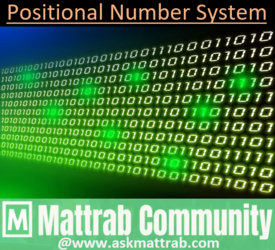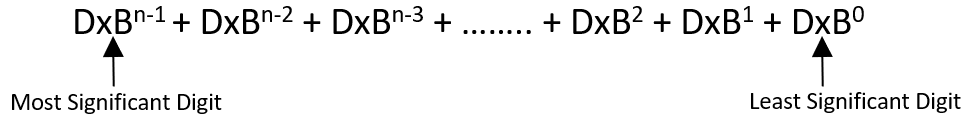# Positional Number System in ComputerMainly, Computer Architecture supports four Positional Number System which are; Decimal, Binary, Octal and hexadecimal. Computer uses decimal number system for user interface whereas the rest of the number system are used for internal processing. The general format of the number system is;where, D=Digits, B is base/radix and n is the position of the number system.
A digit having maximum positional weight is called most significant digit(MSD) whereas a digit having minimum positional weight is least significant digit(LSD).# Decimal Number System
➤ A number system having base(aka Radix) 10 is called Decimal Number System. It consists of 10 digits: 0, 1, 2, 3, 4, 5, 6, 7, 8, and 9. This number is generally used in the computer interface. It can be expressed by using powers of 10 starting with 0 from extreme right digit of the number(i.e., LSD) and increases as we move to left; for non-fractional numbers, the power base starts with -1 and goes on decreasing as move right to decimal.# Binary Number System
A number system having base 2 is called Binary Number System. It consists of 2 digits: 0 and 1. This number is generally used in the internal processing.
An electronic circuit has two state either ON or OFF state. The bit 1 represents ON state and the bit 0 represents OFF state. It can be expressed by using powers of 2 starting with 0 from extreme right digit of the number(i.e., LSD) and increases as we move to left; for non-fractional numbers, the power base starts with -1 and goes on decreasing as move right to decimal.# Octal Number System
➤ A number system having base 8 is called Octal Number System. It consists of 8 digits: 0, 1, 2, 3, 4, 5, 6 and 7. This number is also generally used in the internal processing. It can be expressed by using powers of 8 starting with 0 from extreme right digit of the number(i.e., LSD) and increases as we move to left; for non-fractional numbers, the power base starts with -1 and goes on decreasing as move right to decimal.# Hexadecimal Number System
➤ A number system having base 16 is called Hexadecimal Number System. It consists of 16 digits: 0, 1, 2, 3, 4, 5, 6, 7, 8, 9, A, B, C, D, E, and F. This number is also generally used in computer in memory management. It can be expressed by using powers of 16 starting with 0 from extreme right digit of the number(i.e., LSD) and increases as we move to left; for non-fractional numbers, the power base starts with -1 and goes on decreasing as move right to decimal.